mathtext

path

# mlab¶

## matplotlib.mlab¶

Numerical python functions written for compatability with MATLAB commands with the same names.

### MATLAB compatible functions¶

cohere()
Coherence (normalized cross spectral density)
csd()
Cross spectral density uing Welch’s average periodogram
detrend()
Remove the mean or best fit line from an array
find()
Return the indices where some condition is true;
numpy.nonzero is similar but more general.
griddata()
interpolate irregularly distributed data to a
regular grid.
prctile()
find the percentiles of a sequence
prepca()
Principal Component Analysis
psd()
Power spectral density uing Welch’s average periodogram
rk4()
A 4th order runge kutta integrator for 1D or ND systems
specgram()
Spectrogram (power spectral density over segments of time)

### Miscellaneous functions¶

Functions that don’t exist in MATLAB, but are useful anyway:

cohere_pairs()
Coherence over all pairs. This is not a MATLAB function, but we compute coherence a lot in my lab, and we compute it for a lot of pairs. This function is optimized to do this efficiently by caching the direct FFTs.
rk4()
A 4th order Runge-Kutta ODE integrator in case you ever find yourself stranded without scipy (and the far superior scipy.integrate tools)
contiguous_regions()
return the indices of the regions spanned by some logical mask
cross_from_below()
return the indices where a 1D array crosses a threshold from below
cross_from_above()
return the indices where a 1D array crosses a threshold from above

### record array helper functions¶

A collection of helper methods for numpyrecord arrays

rec2txt()
pretty print a record array
rec2csv()
store record array in CSV file
csv2rec()
import record array from CSV file with type inspection
rec_append_fields()
rec_drop_fields()
drop fields from record array
rec_join()
join two record arrays on sequence of fields
recs_join()
a simple join of multiple recarrays using a single column as a key
rec_groupby()
summarize data by groups (similar to SQL GROUP BY)
rec_summarize()
helper code to filter rec array fields into new fields

For the rec viewer functions(e rec2csv), there are a bunch of Format objects you can pass into the functions that will do things like color negative values red, set percent formatting and scaling, etc.

Example usage:

```r = csv2rec('somefile.csv', checkrows=0)

formatd = dict(
weight = FormatFloat(2),
change = FormatPercent(2),
cost   = FormatThousands(2),
)

rec2excel(r, 'test.xls', formatd=formatd)
rec2csv(r, 'test.csv', formatd=formatd)
scroll = rec2gtk(r, formatd=formatd)

win = gtk.Window()
win.set_size_request(600,800)
win.show_all()
gtk.main()
```

### Deprecated functions¶

The following are deprecated; please import directly from numpy (with care–function signatures may differ):

save()
save ASCII file - use numpy.savetxt
class matplotlib.mlab.FIFOBuffer(*args, **kwargs)

A FIFO queue to hold incoming x, y data in a rotating buffer using numpy arrays under the hood. It is assumed that you will call asarrays much less frequently than you add data to the queue – otherwise another data structure will be faster.

This can be used to support plots where data is added from a real time feed and the plot object wants to grab data from the buffer and plot it to screen less freqeuently than the incoming.

If you set the dataLim attr to BBox (eg matplotlib.Axes.dataLim), the dataLim will be updated as new data come in.

TODO: add a grow method that will extend nmax

Note

mlab seems like the wrong place for this class.

Deprecated since version 1.3: The FIFOBuffer class was deprecated in version 1.3.

Buffer up to nmax points.

Add scalar x and y to the queue.

asarrays()

Return x and y as arrays; their length will be the len of data added or nmax.

last()

Get the last x, y or None. None if no data set.

register(func, N)

Call func every time N events are passed; func signature is func(fifo).

update_datalim_to_current()

Update the datalim in the current data in the fifo.

class matplotlib.mlab.FormatBool
fromstr(s)
toval(x)
class matplotlib.mlab.FormatDate(fmt)
fromstr(x)
toval(x)
class matplotlib.mlab.FormatDatetime(fmt='%Y-%m-%d %H:%M:%S')
fromstr(x)
class matplotlib.mlab.FormatFloat(precision=4, scale=1.0)
fromstr(s)
toval(x)
class matplotlib.mlab.FormatFormatStr(fmt)
tostr(x)
class matplotlib.mlab.FormatInt
fromstr(s)
tostr(x)
toval(x)
class matplotlib.mlab.FormatMillions(precision=4)
class matplotlib.mlab.FormatObj
fromstr(s)
tostr(x)
toval(x)
class matplotlib.mlab.FormatPercent(precision=4)
class matplotlib.mlab.FormatString
tostr(x)
class matplotlib.mlab.FormatThousands(precision=4)
class matplotlib.mlab.PCA(a)

compute the SVD of a and store data for PCA. Use project to project the data onto a reduced set of dimensions

Inputs:

a: a numobservations x numdims array

Attrs:

a a centered unit sigma version of input a

numrows, numcols: the dimensions of a

mu : a numdims array of means of a

sigma : a numdims array of atandard deviation of a

fracs : the proportion of variance of each of the principal components

Wt : the weight vector for projecting a numdims point or array into PCA space

Y : a projected into PCA space

center(x)

center the data using the mean and sigma from training set a

project(x, minfrac=0.0)

project x onto the principle axes, dropping any axes where fraction of variance<minfrac

matplotlib.mlab.amap(function, sequence[, sequence, ...]) → array.

Works like map(), but it returns an array. This is just a convenient shorthand for numpy.array(map(...)).

Return the representation of a number in any given base.

matplotlib.mlab.binary_repr(number, max_length=1025)

Return the binary representation of the input number as a string.

This is more efficient than using base_repr() with base 2.

Increase the value of max_length for very large numbers. Note that on 32-bit machines, 2**1023 is the largest integer power of 2 which can be converted to a Python float.

matplotlib.mlab.bivariate_normal(X, Y, sigmax=1.0, sigmay=1.0, mux=0.0, muy=0.0, sigmaxy=0.0)

Bivariate Gaussian distribution for equal shape X, Y.

See bivariate normal at mathworld.

matplotlib.mlab.center_matrix(M, dim=0)

Return the matrix M with each row having zero mean and unit std.

If dim = 1 operate on columns instead of rows. (dim is opposite to the numpy axis kwarg.)

matplotlib.mlab.cohere(x, y, NFFT=256, Fs=2, detrend=<function detrend_none at 0x256c938>, window=<function window_hanning at 0x256c6e0>, noverlap=0, pad_to=None, sides='default', scale_by_freq=None)

The coherence between x and y. Coherence is the normalized cross spectral density: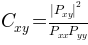x, y
Array or sequence containing the data

Keyword arguments:

NFFT: integer
The number of data points used in each block for the FFT. Must be even; a power 2 is most efficient. The default value is 256. This should NOT be used to get zero padding, or the scaling of the result will be incorrect. Use pad_to for this instead.
Fs: scalar
The sampling frequency (samples per time unit). It is used to calculate the Fourier frequencies, freqs, in cycles per time unit. The default value is 2.
detrend: callable
The function applied to each segment before fft-ing, designed to remove the mean or linear trend. Unlike in MATLAB, where the detrend parameter is a vector, in matplotlib is it a function. The pylab module defines detrend_none(), detrend_mean(), and detrend_linear(), but you can use a custom function as well.
window: callable or ndarray
A function or a vector of length NFFT. To create window vectors see window_hanning(), window_none(), numpy.blackman(), numpy.hamming(), numpy.bartlett(), scipy.signal(), scipy.signal.get_window(), etc. The default is window_hanning(). If a function is passed as the argument, it must take a data segment as an argument and return the windowed version of the segment.
The number of points to which the data segment is padded when performing the FFT. This can be different from NFFT, which specifies the number of data points used. While not increasing the actual resolution of the psd (the minimum distance between resolvable peaks), this can give more points in the plot, allowing for more detail. This corresponds to the n parameter in the call to fft(). The default is None, which sets pad_to equal to NFFT
sides: [ ‘default’ | ‘onesided’ | ‘twosided’ ]
Specifies which sides of the PSD to return. Default gives the default behavior, which returns one-sided for real data and both for complex data. ‘onesided’ forces the return of a one-sided PSD, while ‘twosided’ forces two-sided.
scale_by_freq: boolean
Specifies whether the resulting density values should be scaled by the scaling frequency, which gives density in units of Hz^-1. This allows for integration over the returned frequency values. The default is True for MATLAB compatibility.
noverlap: integer
The number of points of overlap between blocks. The default value is 0 (no overlap).

The return value is the tuple (Cxy, f), where f are the frequencies of the coherence vector. For cohere, scaling the individual densities by the sampling frequency has no effect, since the factors cancel out.

psd() and csd()
For information about the methods used to compute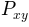,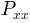and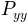.
matplotlib.mlab.cohere_pairs(X, ij, NFFT=256, Fs=2, detrend=<function detrend_none at 0x256c938>, window=<function window_hanning at 0x256c6e0>, noverlap=0, preferSpeedOverMemory=True, progressCallback=<function donothing_callback at 0x256ce60>, returnPxx=False)

Call signature:

```Cxy, Phase, freqs = cohere_pairs( X, ij, ...)
```

Compute the coherence and phase for all pairs ij, in X.

X is a numSamples * numCols array

ij is a list of tuples. Each tuple is a pair of indexes into the columns of X for which you want to compute coherence. For example, if X has 64 columns, and you want to compute all nonredundant pairs, define ij as:

```ij = []
for i in range(64):
for j in range(i+1,64):
ij.append( (i,j) )
```

preferSpeedOverMemory is an optional bool. Defaults to true. If False, limits the caching by only making one, rather than two, complex cache arrays. This is useful if memory becomes critical. Even when preferSpeedOverMemory is False, cohere_pairs() will still give significant performace gains over calling cohere() for each pair, and will use subtantially less memory than if preferSpeedOverMemory is True. In my tests with a 43000,64 array over all nonredundant pairs, preferSpeedOverMemory = True delivered a 33% performance boost on a 1.7GHZ Athlon with 512MB RAM compared with preferSpeedOverMemory = False. But both solutions were more than 10x faster than naively crunching all possible pairs through cohere().

Returns:

```(Cxy, Phase, freqs)
```

where:

• Cxy: dictionary of (i, j) tuples -> coherence vector for that pair. I.e., Cxy[(i,j) = cohere(X[:,i], X[:,j]). Number of dictionary keys is len(ij).

• Phase: dictionary of phases of the cross spectral density at each frequency for each pair. Keys are (i, j).

• freqs: vector of frequencies, equal in length to either the

coherence or phase vectors for any (i, j) key.

e.g., to make a coherence Bode plot:

```subplot(211)
plot( freqs, Cxy[(12,19)])
subplot(212)
plot( freqs, Phase[(12,19)])
```

For a large number of pairs, cohere_pairs() can be much more efficient than just calling cohere() for each pair, because it caches most of the intensive computations. If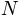is the number of pairs, this function is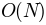for most of the heavy lifting, whereas calling cohere for each pair is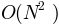. However, because of the caching, it is also more memory intensive, making 2 additional complex arrays with approximately the same number of elements as X.

See test/cohere_pairs_test.py in the src tree for an example script that shows that this cohere_pairs() and cohere() give the same results for a given pair.

psd()
For information about the methods used to compute,and.

return a list of (ind0, ind1) such that mask[ind0:ind1].all() is True and we cover all such regions

TODO: this is a pure python implementation which probably has a much faster numpy impl

matplotlib.mlab.cross_from_above(x, threshold)

return the indices into x where x crosses some threshold from below, eg the i’s where:

```x[i-1]>threshold and x[i]<=threshold
```

matplotlib.mlab.cross_from_below(x, threshold)

return the indices into x where x crosses some threshold from below, eg the i’s where:

```x[i-1]<threshold and x[i]>=threshold
```

Example code:

```import matplotlib.pyplot as plt

t = np.arange(0.0, 2.0, 0.1)
s = np.sin(2*np.pi*t)

fig = plt.figure()
ax.plot(t, s, '-o')
ax.axhline(0.5)
ax.axhline(-0.5)

ind = cross_from_below(s, 0.5)
ax.vlines(t[ind], -1, 1)

ind = cross_from_above(s, -0.5)
ax.vlines(t[ind], -1, 1)

plt.show()
```

matplotlib.mlab.csd(x, y, NFFT=256, Fs=2, detrend=<function detrend_none at 0x256c938>, window=<function window_hanning at 0x256c6e0>, noverlap=0, pad_to=None, sides='default', scale_by_freq=None)

The cross power spectral density by Welch’s average periodogram method. The vectors x and y are divided into NFFT length blocks. Each block is detrended by the function detrend and windowed by the function window. noverlap gives the length of the overlap between blocks. The product of the direct FFTs of x and y are averaged over each segment to compute Pxy, with a scaling to correct for power loss due to windowing.

If len(x) < NFFT or len(y) < NFFT, they will be zero padded to NFFT.

x, y
Array or sequence containing the data

Keyword arguments:

NFFT: integer
The number of data points used in each block for the FFT. Must be even; a power 2 is most efficient. The default value is 256. This should NOT be used to get zero padding, or the scaling of the result will be incorrect. Use pad_to for this instead.
Fs: scalar
The sampling frequency (samples per time unit). It is used to calculate the Fourier frequencies, freqs, in cycles per time unit. The default value is 2.
detrend: callable
The function applied to each segment before fft-ing, designed to remove the mean or linear trend. Unlike in MATLAB, where the detrend parameter is a vector, in matplotlib is it a function. The pylab module defines detrend_none(), detrend_mean(), and detrend_linear(), but you can use a custom function as well.
window: callable or ndarray
A function or a vector of length NFFT. To create window vectors see window_hanning(), window_none(), numpy.blackman(), numpy.hamming(), numpy.bartlett(), scipy.signal(), scipy.signal.get_window(), etc. The default is window_hanning(). If a function is passed as the argument, it must take a data segment as an argument and return the windowed version of the segment.
The number of points to which the data segment is padded when performing the FFT. This can be different from NFFT, which specifies the number of data points used. While not increasing the actual resolution of the psd (the minimum distance between resolvable peaks), this can give more points in the plot, allowing for more detail. This corresponds to the n parameter in the call to fft(). The default is None, which sets pad_to equal to NFFT
sides: [ ‘default’ | ‘onesided’ | ‘twosided’ ]
Specifies which sides of the PSD to return. Default gives the default behavior, which returns one-sided for real data and both for complex data. ‘onesided’ forces the return of a one-sided PSD, while ‘twosided’ forces two-sided.
scale_by_freq: boolean
Specifies whether the resulting density values should be scaled by the scaling frequency, which gives density in units of Hz^-1. This allows for integration over the returned frequency values. The default is True for MATLAB compatibility.
noverlap: integer
The number of points of overlap between blocks. The default value is 0 (no overlap).

Returns the tuple (Pxy, freqs).

Refs:
Bendat & Piersol – Random Data: Analysis and Measurement Procedures, John Wiley & Sons (1986)
matplotlib.mlab.csv2rec(fname, comments='#', skiprows=0, checkrows=0, delimiter=', ', converterd=None, names=None, missing='', missingd=None, use_mrecords=False, dayfirst=False, yearfirst=False)

Load data from comma/space/tab delimited file in fname into a numpy record array and return the record array.

If names is None, a header row is required to automatically assign the recarray names. The headers will be lower cased, spaces will be converted to underscores, and illegal attribute name characters removed. If names is not None, it is a sequence of names to use for the column names. In this case, it is assumed there is no header row.

• fname: can be a filename or a file handle. Support for gzipped files is automatic, if the filename ends in ‘.gz’

• comments: the character used to indicate the start of a comment in the file, or None to switch off the removal of comments

• skiprows: is the number of rows from the top to skip

• checkrows: is the number of rows to check to validate the column data type. When set to zero all rows are validated.

• converterd: if not None, is a dictionary mapping column number or munged column name to a converter function.

• names: if not None, is a list of header names. In this case, no header will be read from the file

• missingd is a dictionary mapping munged column names to field values which signify that the field does not contain actual data and should be masked, e.g., ‘0000-00-00’ or ‘unused’

• missing: a string whose value signals a missing field regardless of the column it appears in

• use_mrecords: if True, return an mrecords.fromrecords record array if any of the data are missing

• dayfirst: default is False so that MM-DD-YY has precedence over DD-MM-YY. See http://labix.org/python-dateutil#head-b95ce2094d189a89f80f5ae52a05b4ab7b41af47 for further information.

• yearfirst: default is False so that MM-DD-YY has precedence over YY-MM-DD. See http://labix.org/python-dateutil#head-b95ce2094d189a89f80f5ae52a05b4ab7b41af47 for further information.

If no rows are found, None is returned – see examples/loadrec.py

matplotlib.mlab.csvformat_factory(format)
matplotlib.mlab.demean(x, axis=0)

Return x minus its mean along the specified axis

matplotlib.mlab.detrend(x, key=None)
matplotlib.mlab.detrend_linear(y)

Return y minus best fit line; ‘linear’ detrending

matplotlib.mlab.detrend_mean(x)

Return x minus the mean(x)

matplotlib.mlab.detrend_none(x)

Return x: no detrending

matplotlib.mlab.dist(x, y)

Return the distance between two points.

matplotlib.mlab.dist_point_to_segment(p, s0, s1)

Get the distance of a point to a segment.

p, s0, s1 are xy sequences
matplotlib.mlab.distances_along_curve(X)

Computes the distance between a set of successive points in N dimensions.

Where X is an M x N array or matrix. The distances between successive rows is computed. Distance is the standard Euclidean distance.

matplotlib.mlab.donothing_callback(*args)
matplotlib.mlab.entropy(y, bins)

Return the entropy of the data in y.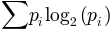where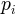is the probability of observing y in the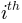bin of bins. bins can be a number of bins or a range of bins; see numpy.histogram().

Compare S with analytic calculation for a Gaussian:

```x = mu + sigma * randn(200000)
Sanalytic = 0.5 * ( 1.0 + log(2*pi*sigma**2.0) )
```
matplotlib.mlab.exp_safe(x)

Compute exponentials which safely underflow to zero.

Slow, but convenient to use. Note that numpy provides proper floating point exception handling with access to the underlying hardware.

matplotlib.mlab.fftsurr(x, detrend=<function detrend_none at 0x256c938>, window=<function window_none at 0x256c758>)

Compute an FFT phase randomized surrogate of x.

matplotlib.mlab.find(condition)

Return the indices where ravel(condition) is true

matplotlib.mlab.frange([start], stop[, step, keywords]) → array of floats

Return a numpy ndarray containing a progression of floats. Similar to numpy.arange(), but defaults to a closed interval.

frange(x0, x1) returns [x0, x0+1, x0+2, ..., x1]; start defaults to 0, and the endpoint is included. This behavior is different from that of range() and numpy.arange(). This is deliberate, since frange() will probably be more useful for generating lists of points for function evaluation, and endpoints are often desired in this use. The usual behavior of range() can be obtained by setting the keyword closed = 0, in this case, frange() basically becomes :func:numpy.arange`.

When step is given, it specifies the increment (or decrement). All arguments can be floating point numbers.

frange(x0,x1,d) returns [x0,x0+d,x0+2d,...,xfin] where xfin <= x1.

frange() can also be called with the keyword npts. This sets the number of points the list should contain (and overrides the value step might have been given). numpy.arange() doesn’t offer this option.

Examples:

```>>> frange(3)
array([ 0.,  1.,  2.,  3.])
>>> frange(3,closed=0)
array([ 0.,  1.,  2.])
>>> frange(1,6,2)
array([1, 3, 5])   or 1,3,5,7, depending on floating point vagueries
>>> frange(1,6.5,npts=5)
array([ 1.   ,  2.375,  3.75 ,  5.125,  6.5  ])
```
matplotlib.mlab.get_formatd(r, formatd=None)

build a formatd guaranteed to have a key for every dtype name

matplotlib.mlab.get_sparse_matrix(M, N, frac=0.1)

Return a M x N sparse matrix with frac elements randomly filled.

matplotlib.mlab.get_xyz_where(Z, Cond)

Z and Cond are M x N matrices. Z are data and Cond is a boolean matrix where some condition is satisfied. Return value is (x, y, z) where x and y are the indices into Z and z are the values of Z at those indices. x, y, and z are 1D arrays.

matplotlib.mlab.griddata(x, y, z, xi, yi, interp='nn')

zi = griddata(x,y,z,xi,yi) fits a surface of the form z = f*(*x, y) to the data in the (usually) nonuniformly spaced vectors (x, y, z). griddata() interpolates this surface at the points specified by (xi, yi) to produce zi. xi and yi must describe a regular grid, can be either 1D or 2D, but must be monotonically increasing.

A masked array is returned if any grid points are outside convex hull defined by input data (no extrapolation is done).

If interp keyword is set to ‘nn‘ (default), uses natural neighbor interpolation based on Delaunay triangulation. By default, this algorithm is provided by the matplotlib.delaunay package, written by Robert Kern. The triangulation algorithm in this package is known to fail on some nearly pathological cases. For this reason, a separate toolkit (mpl_tookits.natgrid) has been created that provides a more robust algorithm fof triangulation and interpolation. This toolkit is based on the NCAR natgrid library, which contains code that is not redistributable under a BSD-compatible license. When installed, this function will use the mpl_toolkits.natgrid algorithm, otherwise it will use the built-in matplotlib.delaunay package.

If the interp keyword is set to ‘linear‘, then linear interpolation is used instead of natural neighbor. In this case, the output grid is assumed to be regular with a constant grid spacing in both the x and y directions. For regular grids with nonconstant grid spacing, you must use natural neighbor interpolation. Linear interpolation is only valid if matplotlib.delaunay package is used - mpl_tookits.natgrid only provides natural neighbor interpolation.

matplotlib.mlab.identity(n, rank=2, dtype='l', typecode=None)

Returns the identity matrix of shape (n, n, ..., n) (rank r).

For ranks higher than 2, this object is simply a multi-index Kronecker delta:

```                    /  1  if i0=i1=...=iR,
id[i0,i1,...,iR] = -|
\  0  otherwise.```

Optionally a dtype (or typecode) may be given (it defaults to ‘l’).

Since rank defaults to 2, this function behaves in the default case (when only n is given) like numpy.identity(n) – but surprisingly, it is much faster.

matplotlib.mlab.inside_poly(points, verts)

points is a sequence of x, y points. verts is a sequence of x, y vertices of a polygon.

Return value is a sequence of indices into points for the points that are inside the polygon.

matplotlib.mlab.is_closed_polygon(X)

Tests whether first and last object in a sequence are the same. These are presumably coordinates on a polygonal curve, in which case this function tests if that curve is closed.

matplotlib.mlab.ispower2(n)

Returns the log base 2 of n if n is a power of 2, zero otherwise.

Note the potential ambiguity if n == 1: 2**0 == 1, interpret accordingly.

matplotlib.mlab.isvector(X)

Like the MATLAB function with the same name, returns True if the supplied numpy array or matrix X looks like a vector, meaning it has a one non-singleton axis (i.e., it can have multiple axes, but all must have length 1, except for one of them).

If you just want to see if the array has 1 axis, use X.ndim == 1.

matplotlib.mlab.l1norm(a)

Return the l1 norm of a, flattened out.

Implemented as a separate function (not a call to norm() for speed).

matplotlib.mlab.l2norm(a)

Return the l2 norm of a, flattened out.

Implemented as a separate function (not a call to norm() for speed).

matplotlib.mlab.less_simple_linear_interpolation(x, y, xi, extrap=False)

This function provides simple (but somewhat less so than cbook.simple_linear_interpolation()) linear interpolation. simple_linear_interpolation() will give a list of point between a start and an end, while this does true linear interpolation at an arbitrary set of points.

This is very inefficient linear interpolation meant to be used only for a small number of points in relatively non-intensive use cases. For real linear interpolation, use scipy.

matplotlib.mlab.levypdf(x, gamma, alpha)

Returm the levy pdf evaluated at x for params gamma, alpha

matplotlib.mlab.log2(x, ln2=0.6931471805599453)

Return the log(x) in base 2.

This is a _slow_ function but which is guaranteed to return the correct integer value if the input is an integer exact power of 2.

matplotlib.mlab.logspace(xmin, xmax, N)
matplotlib.mlab.longest_contiguous_ones(x)

Return the indices of the longest stretch of contiguous ones in x, assuming x is a vector of zeros and ones. If there are two equally long stretches, pick the first.

matplotlib.mlab.longest_ones(x)

alias for longest_contiguous_ones

matplotlib.mlab.movavg(x, n)

Compute the len(n) moving average of x.

matplotlib.mlab.norm_flat(a, p=2)

norm(a,p=2) -> l-p norm of a.flat

Return the l-p norm of a, considered as a flat array. This is NOT a true matrix norm, since arrays of arbitrary rank are always flattened.

p can be a number or the string ‘Infinity’ to get the L-infinity norm.

matplotlib.mlab.normpdf(x, *args)

Return the normal pdf evaluated at x; args provides mu, sigma

matplotlib.mlab.offset_line(y, yerr)

Offsets an array y by +/- an error and returns a tuple (y - err, y + err).

The error term can be:

• A scalar. In this case, the returned tuple is obvious.

• A vector of the same length as y. The quantities y +/- err are computed component-wise.

• A tuple of length 2. In this case, yerr is the error below y and yerr is error above y. For example:

```from pylab import *
x = linspace(0, 2*pi, num=100, endpoint=True)
y = sin(x)
y_minus, y_plus = mlab.offset_line(y, 0.1)
plot(x, y)
fill_between(x, ym, y2=yp)
show()
```
matplotlib.mlab.path_length(X)

Computes the distance travelled along a polygonal curve in N dimensions.

Where X is an M x N array or matrix. Returns an array of length M consisting of the distance along the curve at each point (i.e., the rows of X).

matplotlib.mlab.poly_below(xmin, xs, ys)

Given a sequence of xs and ys, return the vertices of a polygon that has a horizontal base at xmin and an upper bound at the ys. xmin is a scalar.

Intended for use with matplotlib.axes.Axes.fill(), eg:

```xv, yv = poly_below(0, x, y)
ax.fill(xv, yv)
```
matplotlib.mlab.poly_between(x, ylower, yupper)

Given a sequence of x, ylower and yupper, return the polygon that fills the regions between them. ylower or yupper can be scalar or iterable. If they are iterable, they must be equal in length to x.

Return value is x, y arrays for use with matplotlib.axes.Axes.fill().

matplotlib.mlab.prctile(x, p=(0.0, 25.0, 50.0, 75.0, 100.0))

Return the percentiles of x. p can either be a sequence of percentile values or a scalar. If p is a sequence, the ith element of the return sequence is the p*(i)-th percentile of *x. If p is a scalar, the largest value of x less than or equal to the p percentage point in the sequence is returned.

matplotlib.mlab.prctile_rank(x, p)

Return the rank for each element in x, return the rank 0..len(p). e.g., if p = (25, 50, 75), the return value will be a len(x) array with values in [0,1,2,3] where 0 indicates the value is less than the 25th percentile, 1 indicates the value is >= the 25th and < 50th percentile, ... and 3 indicates the value is above the 75th percentile cutoff.

p is either an array of percentiles in [0..100] or a scalar which indicates how many quantiles of data you want ranked.

matplotlib.mlab.prepca(P, frac=0)

Compute the principal components of P. P is a (numVars, numObs) array. frac is the minimum fraction of variance that a component must contain to be included.

Return value is a tuple of the form (Pcomponents, Trans, fracVar) where:

• Pcomponents : a (numVars, numObs) array

• Trans : the weights matrix, ie, Pcomponents = Trans *

P

• fracVar : the fraction of the variance accounted for by each

component returned

A similar function of the same name was in the MATLAB R13 Neural Network Toolbox but is not found in later versions; its successor seems to be called “processpcs”.

matplotlib.mlab.psd(x, NFFT=256, Fs=2, detrend=<function detrend_none at 0x256c938>, window=<function window_hanning at 0x256c6e0>, noverlap=0, pad_to=None, sides='default', scale_by_freq=None)

The power spectral density by Welch’s average periodogram method. The vector x is divided into NFFT length blocks. Each block is detrended by the function detrend and windowed by the function window. noverlap gives the length of the overlap between blocks. The absolute(fft(block))**2 of each segment are averaged to compute Pxx, with a scaling to correct for power loss due to windowing.

If len(x) < NFFT, it will be zero padded to NFFT.

x
Array or sequence containing the data

Keyword arguments:

NFFT: integer
The number of data points used in each block for the FFT. Must be even; a power 2 is most efficient. The default value is 256. This should NOT be used to get zero padding, or the scaling of the result will be incorrect. Use pad_to for this instead.
Fs: scalar
The sampling frequency (samples per time unit). It is used to calculate the Fourier frequencies, freqs, in cycles per time unit. The default value is 2.
detrend: callable
The function applied to each segment before fft-ing, designed to remove the mean or linear trend. Unlike in MATLAB, where the detrend parameter is a vector, in matplotlib is it a function. The pylab module defines detrend_none(), detrend_mean(), and detrend_linear(), but you can use a custom function as well.
window: callable or ndarray
A function or a vector of length NFFT. To create window vectors see window_hanning(), window_none(), numpy.blackman(), numpy.hamming(), numpy.bartlett(), scipy.signal(), scipy.signal.get_window(), etc. The default is window_hanning(). If a function is passed as the argument, it must take a data segment as an argument and return the windowed version of the segment.
The number of points to which the data segment is padded when performing the FFT. This can be different from NFFT, which specifies the number of data points used. While not increasing the actual resolution of the psd (the minimum distance between resolvable peaks), this can give more points in the plot, allowing for more detail. This corresponds to the n parameter in the call to fft(). The default is None, which sets pad_to equal to NFFT
sides: [ ‘default’ | ‘onesided’ | ‘twosided’ ]
Specifies which sides of the PSD to return. Default gives the default behavior, which returns one-sided for real data and both for complex data. ‘onesided’ forces the return of a one-sided PSD, while ‘twosided’ forces two-sided.
scale_by_freq: boolean
Specifies whether the resulting density values should be scaled by the scaling frequency, which gives density in units of Hz^-1. This allows for integration over the returned frequency values. The default is True for MATLAB compatibility.
noverlap: integer
The number of points of overlap between blocks. The default value is 0 (no overlap).

Returns the tuple (Pxx, freqs).

Refs:

Bendat & Piersol – Random Data: Analysis and Measurement Procedures, John Wiley & Sons (1986)
matplotlib.mlab.quad2cubic(q0x, q0y, q1x, q1y, q2x, q2y)

Converts a quadratic Bezier curve to a cubic approximation.

The inputs are the x and y coordinates of the three control points of a quadratic curve, and the output is a tuple of x and y coordinates of the four control points of the cubic curve.

matplotlib.mlab.rec2csv(r, fname, delimiter=', ', formatd=None, missing='', missingd=None, withheader=True)

Save the data from numpy recarray r into a comma-/space-/tab-delimited file. The record array dtype names will be used for column headers.

fname: can be a filename or a file handle. Support for gzipped
files is automatic, if the filename ends in ‘.gz’
names in the first row

for formatd type FormatFloat, we override the precision to store full precision floats in the CSV file

csv2rec()
For information about missing and missingd, which can be used to fill in masked values into your CSV file.

Returns a textual representation of a record array.

r: numpy recarray

precision: number of decimal places to use for floats.
Set to an integer to apply to all floats. Set to a list of integers to apply precision individually. Precision for non-floats is simply ignored.

fields : if not None, a list of field names to print. fields can be a list of strings like [‘field1’, ‘field2’] or a single comma separated string like ‘field1,field2’

Example:

```precision=[0,2,3]
```

Output:

```ID    Price   Return
ABC   12.54    0.234
XYZ    6.32   -0.076```
matplotlib.mlab.rec_append_fields(rec, names, arrs, dtypes=None)

Return a new record array with field names populated with data from arrays in arrs. If appending a single field, then names, arrs and dtypes do not have to be lists. They can just be the values themselves.

matplotlib.mlab.rec_drop_fields(rec, names)

Return a new numpy record array with fields in names dropped.

matplotlib.mlab.rec_groupby(r, groupby, stats)

r is a numpy record array

groupby is a sequence of record array attribute names that together form the grouping key. eg (‘date’, ‘productcode’)

stats is a sequence of (attr, func, outname) tuples which will call x = func(attr) and assign x to the record array output with attribute outname. For example:

```stats = ( ('sales', len, 'numsales'), ('sales', np.mean, 'avgsale') )
```

Return record array has dtype names for each attribute name in the the groupby argument, with the associated group values, and for each outname name in the stats argument, with the associated stat summary output.

matplotlib.mlab.rec_join(key, r1, r2, jointype='inner', defaults=None, r1postfix='1', r2postfix='2')

Join record arrays r1 and r2 on key; key is a tuple of field names – if key is a string it is assumed to be a single attribute name. If r1 and r2 have equal values on all the keys in the key tuple, then their fields will be merged into a new record array containing the intersection of the fields of r1 and r2.

r1 (also r2) must not have any duplicate keys.

The jointype keyword can be ‘inner’, ‘outer’, ‘leftouter’. To do a rightouter join just reverse r1 and r2.

The defaults keyword is a dictionary filled with {column_name:default_value} pairs.

The keywords r1postfix and r2postfix are postfixed to column names (other than keys) that are both in r1 and r2.

matplotlib.mlab.rec_keep_fields(rec, names)

Return a new numpy record array with only fields listed in names

matplotlib.mlab.rec_summarize(r, summaryfuncs)

r is a numpy record array

summaryfuncs is a list of (attr, func, outname) tuples which will apply func to the the array r*[attr] and assign the output to a new attribute name *outname. The returned record array is identical to r, with extra arrays for each element in summaryfuncs.

matplotlib.mlab.recs_join(key, name, recs, jointype='outer', missing=0.0, postfixes=None)

Join a sequence of record arrays on single column key.

This function only joins a single column of the multiple record arrays

key
is the column name that acts as a key
name
is the name of the column that we want to join
recs
is a list of record arrays to join
jointype
is a string ‘inner’ or ‘outer’
missing
is what any missing field is replaced by
postfixes
if not None, a len recs sequence of postfixes

returns a record array with columns [rowkey, name0, name1, ... namen-1]. or if postfixes [PF0, PF1, ..., PFN-1] are supplied, [rowkey, namePF0, namePF1, ... namePFN-1].

Example:

```r = recs_join("date", "close", recs=[r0, r1], missing=0.)
```
matplotlib.mlab.rk4(derivs, y0, t)

Integrate 1D or ND system of ODEs using 4-th order Runge-Kutta. This is a toy implementation which may be useful if you find yourself stranded on a system w/o scipy. Otherwise use scipy.integrate().

y0
initial state vector
t
sample times
derivs
returns the derivative of the system and has the signature dy = derivs(yi, ti)

Example 1

```## 2D system

def derivs6(x,t):
d1 =  x + 2*x
d2 =  -3*x + 4*x
return (d1, d2)
dt = 0.0005
t = arange(0.0, 2.0, dt)
y0 = (1,2)
yout = rk4(derivs6, y0, t)
```

Example 2:

```## 1D system
alpha = 2
def derivs(x,t):
return -alpha*x + exp(-t)

y0 = 1
yout = rk4(derivs, y0, t)
```

If you have access to scipy, you should probably be using the scipy.integrate tools rather than this function.

matplotlib.mlab.rms_flat(a)

Return the root mean square of all the elements of a, flattened out.

matplotlib.mlab.safe_isinf(x)

numpy.isinf() for arbitrary types

matplotlib.mlab.safe_isnan(x)

numpy.isnan() for arbitrary types

matplotlib.mlab.segments_intersect(s1, s2)

Return True if s1 and s2 intersect. s1 and s2 are defined as:

```s1: (x1, y1), (x2, y2)
s2: (x3, y3), (x4, y4)```
matplotlib.mlab.slopes(x, y)

slopes() calculates the slope y‘(x)

The slope is estimated using the slope obtained from that of a parabola through any three consecutive points.

This method should be superior to that described in the appendix of A CONSISTENTLY WELL BEHAVED METHOD OF INTERPOLATION by Russel W. Stineman (Creative Computing July 1980) in at least one aspect:

Circles for interpolation demand a known aspect ratio between x- and y-values. For many functions, however, the abscissa are given in different dimensions, so an aspect ratio is completely arbitrary.

The parabola method gives very similar results to the circle method for most regular cases but behaves much better in special cases.

Norbert Nemec, Institute of Theoretical Physics, University or Regensburg, April 2006 Norbert.Nemec at physik.uni-regensburg.de

(inspired by a original implementation by Halldor Bjornsson, Icelandic Meteorological Office, March 2006 halldor at vedur.is)

matplotlib.mlab.specgram(x, NFFT=256, Fs=2, detrend=<function detrend_none at 0x256c938>, window=<function window_hanning at 0x256c6e0>, noverlap=128, pad_to=None, sides='default', scale_by_freq=None)

Compute a spectrogram of data in x. Data are split into NFFT length segments and the PSD of each section is computed. The windowing function window is applied to each segment, and the amount of overlap of each segment is specified with noverlap.

If x is real (i.e. non-complex) only the spectrum of the positive frequencie is returned. If x is complex then the complete spectrum is returned.

Keyword arguments:

NFFT: integer
The number of data points used in each block for the FFT. Must be even; a power 2 is most efficient. The default value is 256. This should NOT be used to get zero padding, or the scaling of the result will be incorrect. Use pad_to for this instead.
Fs: scalar
The sampling frequency (samples per time unit). It is used to calculate the Fourier frequencies, freqs, in cycles per time unit. The default value is 2.
detrend: callable
The function applied to each segment before fft-ing, designed to remove the mean or linear trend. Unlike in MATLAB, where the detrend parameter is a vector, in matplotlib is it a function. The pylab module defines detrend_none(), detrend_mean(), and detrend_linear(), but you can use a custom function as well.
window: callable or ndarray
A function or a vector of length NFFT. To create window vectors see window_hanning(), window_none(), numpy.blackman(), numpy.hamming(), numpy.bartlett(), scipy.signal(), scipy.signal.get_window(), etc. The default is window_hanning(). If a function is passed as the argument, it must take a data segment as an argument and return the windowed version of the segment.
The number of points to which the data segment is padded when performing the FFT. This can be different from NFFT, which specifies the number of data points used. While not increasing the actual resolution of the psd (the minimum distance between resolvable peaks), this can give more points in the plot, allowing for more detail. This corresponds to the n parameter in the call to fft(). The default is None, which sets pad_to equal to NFFT
sides: [ ‘default’ | ‘onesided’ | ‘twosided’ ]
Specifies which sides of the PSD to return. Default gives the default behavior, which returns one-sided for real data and both for complex data. ‘onesided’ forces the return of a one-sided PSD, while ‘twosided’ forces two-sided.
scale_by_freq: boolean
Specifies whether the resulting density values should be scaled by the scaling frequency, which gives density in units of Hz^-1. This allows for integration over the returned frequency values. The default is True for MATLAB compatibility.
noverlap: integer
The number of points of overlap between blocks. The default value is 128.

Returns a tuple (Pxx, freqs, t):

• Pxx: 2-D array, columns are the periodograms of successive segments
• freqs: 1-D array of frequencies corresponding to the rows in Pxx
• t: 1-D array of times corresponding to midpoints of segments.

psd()
psd() differs in the default overlap; in returning the mean of the segment periodograms; and in not returning times.
matplotlib.mlab.stineman_interp(xi, x, y, yp=None)

Given data vectors x and y, the slope vector yp and a new abscissa vector xi, the function stineman_interp() uses Stineman interpolation to calculate a vector yi corresponding to xi.

Here’s an example that generates a coarse sine curve, then interpolates over a finer abscissa:

```x = linspace(0,2*pi,20);  y = sin(x); yp = cos(x)
xi = linspace(0,2*pi,40);
yi = stineman_interp(xi,x,y,yp);
plot(x,y,'o',xi,yi)
```

The interpolation method is described in the article A CONSISTENTLY WELL BEHAVED METHOD OF INTERPOLATION by Russell W. Stineman. The article appeared in the July 1980 issue of Creative Computing with a note from the editor stating that while they were:

not an academic journal but once in a while something serious and original comes in adding that this was “apparently a real solution” to a well known problem.

For yp = None, the routine automatically determines the slopes using the slopes() routine.

x is assumed to be sorted in increasing order.

For values xi[j] < x or xi[j] > x[-1], the routine tries an extrapolation. The relevance of the data obtained from this, of course, is questionable...

Original implementation by Halldor Bjornsson, Icelandic Meteorolocial Office, March 2006 halldor at vedur.is

Completely reworked and optimized for Python by Norbert Nemec, Institute of Theoretical Physics, University or Regensburg, April 2006 Norbert.Nemec at physik.uni-regensburg.de

matplotlib.mlab.vector_lengths(X, P=2.0, axis=None)

Finds the length of a set of vectors in n dimensions. This is like the numpy.norm() function for vectors, but has the ability to work over a particular axis of the supplied array or matrix.

Computes (sum((x_i)^P))^(1/P) for each {x_i} being the elements of X along the given axis. If axis is None, compute over all elements of X.

matplotlib.mlab.window_hanning(x)

return x times the hanning window of len(x)

matplotlib.mlab.window_none(x)

No window function; simply return x